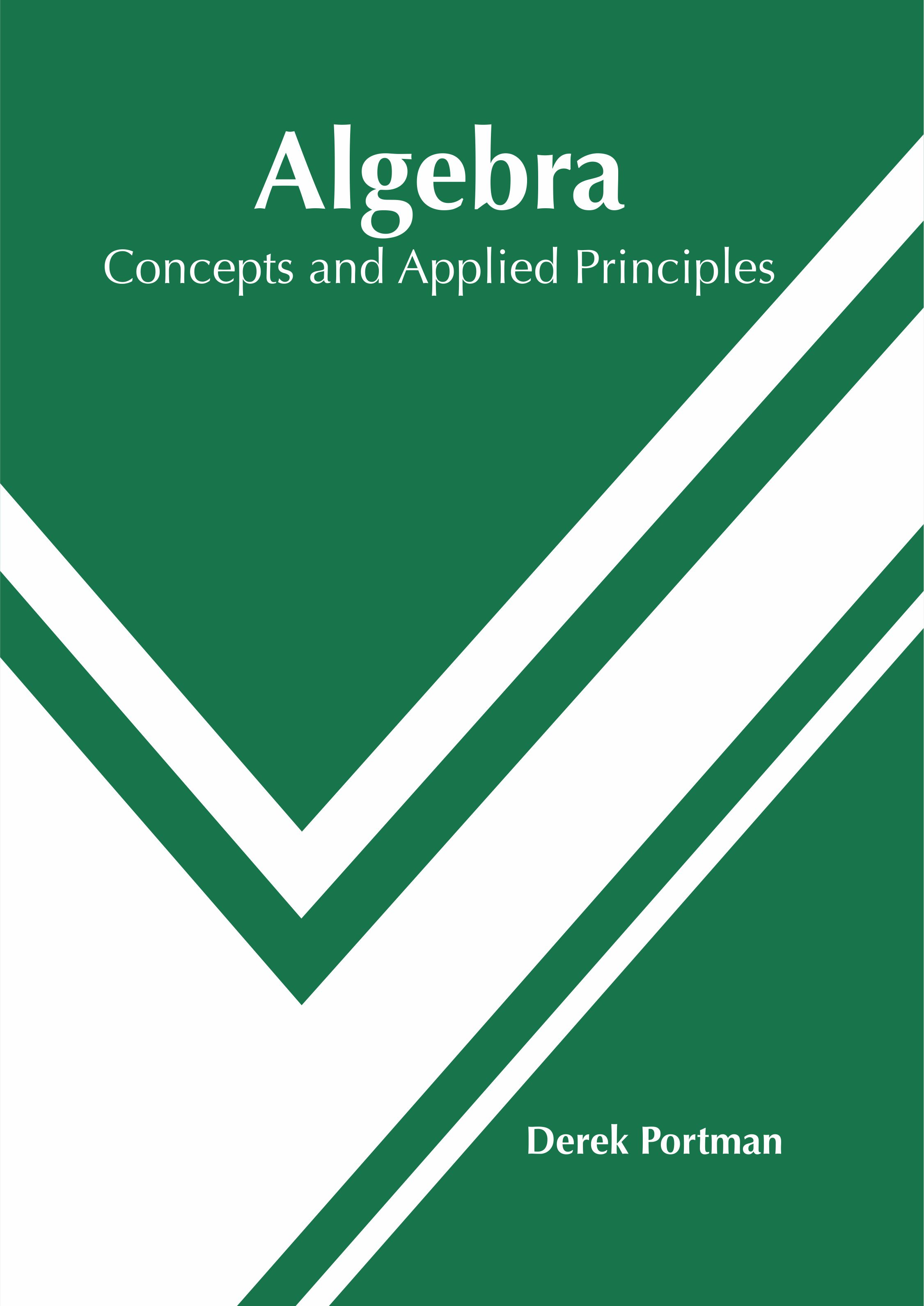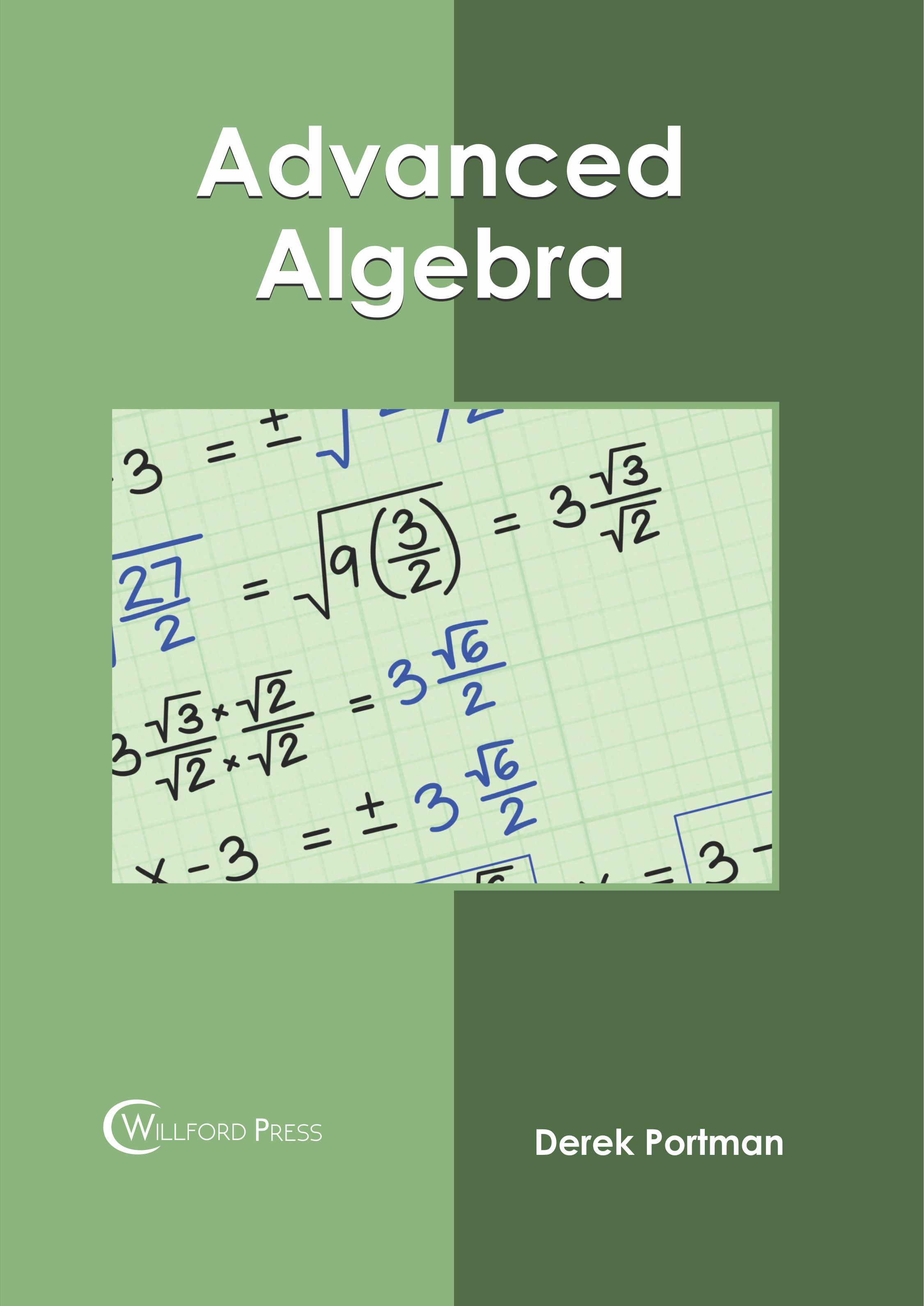BROWSE BY

#### Author

Results 1 - 4 of 4Algebra: Advanced Concepts Author : Derek Portman Subject : Mathematics ISBN :9781632386717 Algebra is an area of mathematics that studies mathematical symbols and their rules of manipulation. It is instrumental in equation solving and in the study of abstractions like rings, groups and fields. Read MoreAlgebra: Concepts and Applied Principles Author : Derek Portman Subject : Mathematics ISBN :9781632386649 Algebra is a fundamental part of mathematics. It deals with the manipulation of mathematical symbols using specific rules. It is divided into the two branches of elementary algebra and abstract algebra. Read MoreAlgebra: Advanced Mathematics Author : Derek Portman Subject : Mathematics ISBN :9781682858257 Algebra is one of the major branches of mathematics which studies mathematical symbols and the rules used for handling these symbols. It deals with numerous concepts such as elementary equations and abstractions Read MoreAdvanced Algebra Author : Derek Portman Subject : Mathematics ISBN :9781682856345 "Algebra is a sub-division of mathematics that involves elementary equation solving and study of abstractions. Advanced algebra or abstract algebra is the study of algebraic structures such as groups, Read More
Results 1 - 4 of 4